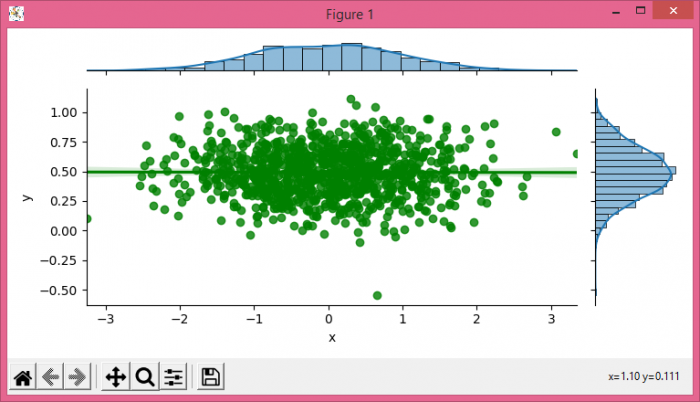# How to change the line color in a Seaborn linear regression jointplot?

MatplotlibPythonData Visualization

#### Python Data Science basics with Numpy, Pandas and Matplotlib

Most Popular

63 Lectures 6 hours

#### Data Visualization using MatPlotLib & Seaborn

11 Lectures 4 hours

#### MatPlotLib with Python

9 Lectures 2.5 hours

To change the line color in seaborn linear regression jointplot, we can use joint_kws in jointplot() method.

## Steps

• Set the figure size and adjust the padding between and around the subplots.
• Create x and y data points using numpy to make a Pandas dataframe.
• Use jointplot() method with joint_kws in the arguments.
• To display the figure, use show() method.

## Example

import seaborn as sns
import numpy as np
from matplotlib import pyplot as plt
import pandas as pd

plt.rcParams["figure.figsize"] = [7.50, 3.50]
plt.rcParams["figure.autolayout"] = True

X = np.random.randn(1000,)
Y = 0.2 * np.random.randn(1000) + 0.5

df = pd.DataFrame(dict(x=X, y=Y))
g = sns.jointplot(x="x", y="y", data=df, kind='reg', height=3.5, joint_kws={'color':'green'})

plt.show()

## Output